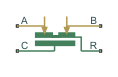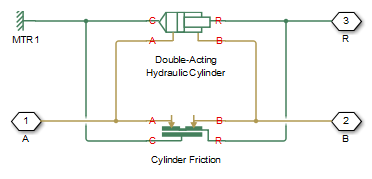# Cylinder Friction

(To be removed) Friction in hydraulic cylinders

The Hydraulics (Isothermal) library will be removed in a future release. Use the Isothermal Liquid library instead. (since R2020a)

•Libraries:
Simscape / Fluids / Hydraulics (Isothermal) / Hydraulic Cylinders

## Description

The Cylinder Friction block simulates friction in the contact between moving bodies in hydraulic cylinders. Use this block as a building block in combination with double- and single-acting cylinders to develop a cylinder model with friction. The block simulates the friction force as a function of relative velocity and pressure, and assumes this force is the sum of the Stribeck, Coulomb, and viscous components. The Coulomb friction force consists of the preload force, which is caused by the seal squeeze during assembly, and the force proportional to the pressure. The sum of the Coulomb and Stribeck friction forces at zero velocity is the breakaway friction force. For more information, see the Translational Friction block reference page.

The block approximates the friction force as

`$F={F}_{C}·\left(1+\left({K}_{brk}-1\right)·\mathrm{exp}\left(-{c}_{v}|v|\right)\right)sign\left(v\right)+{f}_{vfr}·v$`
`${F}_{C}={F}_{pr}+{f}_{cfr}\left({p}_{A}+{p}_{B}\right)$`

where:

 F Friction force FC Coulomb friction Fpr Preload force fcfr Coulomb friction coefficient pA, pB Pressures in cylinder chambers Kbrk Breakaway friction force increase coefficient cv Transition coefficient v Relative velocity in the contact fvfr Viscous friction coefficient

To avoid discontinuity at v = 0, the block introduces a small region, |v| ≤ vth, around zero velocity where the friction force is linearly proportional to velocity

`$F=K·v$`
`$K=\frac{{F}_{C}\left(1+\left({K}_{brk}-1\right)·\mathrm{exp}\left(-{c}_{v}{v}_{th}\right)\right)+{f}_{vfr}·{v}_{th}}{{v}_{th}}$`

where:

 K Proportionality coefficient vth Velocity threshold

R and C are mechanical translational conserving ports associated with the rod and case, respectively. A and B are hydraulic conserving ports that you connect to ports A and B of the cylinder block, as shown in this illustration. The force generated by the block always opposes relative motion between the rod and the case.### Variables

To set the priority and initial target values for the block variables prior to simulation, use the Initial Targets section in the block dialog box or Property Inspector. For more information, see Set Priority and Initial Target for Block Variables.

Nominal values provide a way to specify the expected magnitude of a variable in a model. Using system scaling based on nominal values increases the simulation robustness. Nominal values can come from different sources, one of which is the Nominal Values section in the block dialog box or Property Inspector. For more information, see Modify Nominal Values for a Block Variable.

## Ports

### Conserving

expand all

Hydraulic conserving port connected to the cylinder inlet.

Hydraulic conserving port connected to the cylinder outlet.

Mechanical translational conserving port associated with the cylinder rod.

Mechanical translational conserving port associated with the cylinder clamping structure.

## Parameters

expand all

Preload force caused by the seal squeeze during assembly.

Coulomb friction coefficient. This value defines the proportionality between the Coulomb friction force and the pressure in cylinder chambers.

Friction force increase over the Coulomb friction. The Coulomb friction force, multiplied by this coefficient, is breakaway friction force.

Proportionality coefficient between the viscous friction force and the relative velocity.

Value of the coefficient cv, which the block uses for the approximation of the transition between the breakaway and the Coulomb frictions. Assign this value based on the following considerations: the Stribeck friction component reaches approximately 5% of its steady-state value at velocity `3`/cv, and 2% at velocity `4`/cv, which makes it possible to develop an approximate relationship cv ~= `4`/vmin, where vmin is the relative velocity at which friction force has its minimum value. The default value, `10` s/m, corresponds to a minimum friction at a velocity of about `0.4` m/s.

Small vicinity near zero velocity where the friction force is linearly proportional to the relative velocity. It is recommended that you use values in the range between `1e-6` and `1e-4` m/s.

## Version History

Introduced in R2006b

expand all